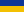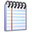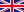Complete analytical description of the fundamental solution of the one parabolic equation with increasing coefficients / Zabolotko, T. O., Ivasyshen, S. D. (2014)web address of the page http://jnas.nbuv.gov.ua/article/UJRN-0000217773  Mathematical and computer modelling. Series: Physical and mathematical sciences   Б - 2020  /Issue (2014, Вип. 10)Заболотько Т. О., Івасишен С. Д.Повне аналітичне описання фундаментального розв'язку одного параболічного рівняння зі зростаючими коефіцієнтами Бібліографічний опис:Заболотько Т. О., Івасишен С. Д. Повне аналітичне описання фундаментального розв'язку одного параболічного рівняння зі зростаючими коефіцієнтами. Математичне та комп'ютерне моделювання. Сер. : Фізико-математичні науки. 2014. Вип. 10. С. 88-98. URL: http://jnas.nbuv.gov.ua/article/UJRN-0000217773Mathematical and computer modelling. Series: Physical and mathematical sciences    /Issue (2014, 10)Zabolotko T. O., Ivasyshen S. D.Complete analytical description of the fundamental solution of the one parabolic equation with increasing coefficientsCite:Zabolotko, T. O., Ivasyshen, S. D. (2014). Complete analytical description of the fundamental solution of the one parabolic equation with increasing coefficients. Mathematical and computer modelling. Series: Physical and mathematical sciences , 10, 88-98. http://jnas.nbuv.gov.ua/article/UJRN-0000217773 [In Ukrainian].

 National Academy of Sciences of UkraineLibrary portal of National Academy of Sciences of Ukraine | LibNAShttp://libnas.nbuv.gov.uaVernadsky National Library of Ukraine (VNLU)Institute of Information Technologies of VNLU+38 (044) 525-36-24libnas@nbuv.gov.uaUkraine, 03039, Kyiv, Holosiivskyi Ave, 3, room 209